# Backward Elimination in Machine Learning

In this page we will learn Backward Elimination in Machine Learning, What is Backward Elimination in Machine Learning?, Steps of Backward Elimination, Need for Backward Elimination: An optimal Multiple Linear Regression model, Steps for Backward Elimination method, Backward Elimination Preparation.

## What is Backward Elimination in Machine Learning?

When developing a machine learning model, backward elimination is a feature selection strategy. It's utilized to get rid of features that don't have much of an impact on the dependent variable or output prediction. In Machine Learning, there are several techniques to construct a model, including:

1. All-in
2. Backward Elimination
3. Forward Selection
4. Bidirectional Elimination
5. Score Comparison

The methods for developing a model in Machine Learning are listed above, however we will only utilize the Backward Elimination approach because it is the fastest.

## Steps of Backward Elimination

The following are the main steps in the backward elimination process:

Step 1: To stay in the model, we must first choose a significance level. (SL = 0.05)
Step 2: Complete the model by include all possible predictors and independent variables.
Step 3: Select the predictor with the highest P-value, for example.

• If P-value >SL, go to step 4.
• Else Finish, and Our model is ready

Step-4: Remove that predictor.
Step-5: Rebuild and fit the model with the remaining variables.

## Need for Backward Elimination: An optimal Multiple Linear Regression model:

We examined and successfully developed our Multiple Linear Regression model in the previous chapter, where we used four independent variables (R&D spend, Administration spend, Marketing spend, and state (dummy variables)) and one dependent variable (R&D spend) (Profit). However, that model is not ideal because we have included all of the independent variables and do not know which independent model has the greatest impact on the forecast.
Unnecessary features add to the model's complexity. As a result, it is preferable to have only the most important elements and keep our model basic in order to achieve the best results. So, in order to improve the model's performance, we'll apply the Backward Elimination approach. This approach is used to improve the MLR model's performance by only including the most important features and excluding the least important ones. Let's put it to work on our MLR model.

## Steps for Backward Elimination method:

We'll utilize the same model that we created in the previous MLR chapter. The whole code for it is as follows:

``````
#importing libraries
import numpy as nm
import matplotlib.pyplot as mtp
import pandas as pd

#importing datasets

#Extracting Independent and dependent Variable
x = data_set.iloc[:, :-1].values
y = data_set.iloc[:, 4].values

#Catgorical data
from sklearn.preprocessing import LabelEncoder, OneHotEncoder
labelencoder_x = LabelEncoder()
x[:, 3] = labelencoder_x.fit_transform(x[:,3])
onehotencoder = OneHotEncoder(categorical_features = )
x = onehotencoder.fit_transform(x).toarray()

#Avoiding the dummy variable trap:
x = x[:, 1:]

#Splitting the dataset into training and test set.
from sklearn.model_selection import train_test_split
x_train, x_test, y_train, y_test= train_test_split(x, y, test_size= 0.2, random_state=0)

#Fitting the MLR model to the training set:
from sklearn.linear_model import LinearRegression
regressor = LinearRegression()
regressor.fit(x_train, y_train)

#Predicting the Test set result;
y_pred = regressor.predict(x_test)

#Checking the score
print('Train Score: ', regressor.score(x_train, y_train))
print('Test Score: ', regressor.score(x_test, y_test))
```
```

From the above code, we got training and test set result as: />

``````
Train Score: 0.9501847627493607
Test Score: 0.9347068473282446
```
```

The difference between both scores is 0.0154.

[ Note: Using the Backward elimination procedure, we will evaluate the effect of features on our model based on this score. ]

## Step 1: Backward Elimination Preparation:

• Importing the library in matrix feature: To begin, we must import the statsmodels.formula.api library, which is used to estimate various statistical models such as the OLS model (Ordinary Least Square). The code for it is as follows:
``````
import statsmodels.api as smf
```
```
• Adding a column to a feature matrix: There is one constant term b0 in our MLR equation (a), however this term is not included in our matrix of features, therefore we must manually add it. A column with the values x0 = 1 connected with the constant term b0 will be added.
To do so, we'll utilize the Numpy library's append function (nm, which we've already imported into our code) and assign a value of 1. The code can be found below.
``````
x = nm.append(arr = nm.ones((50,1)).astype(int), values=x, axis = 1)
```
```

Here we have used axis =1, as we wanted to add a column. For adding a row, we can use axis =0.

Output: Executing the preceding line of code will add a new column to our matrix of features, with all values equal to one. We may examine it by selecting the x dataset from the variable explorer menu.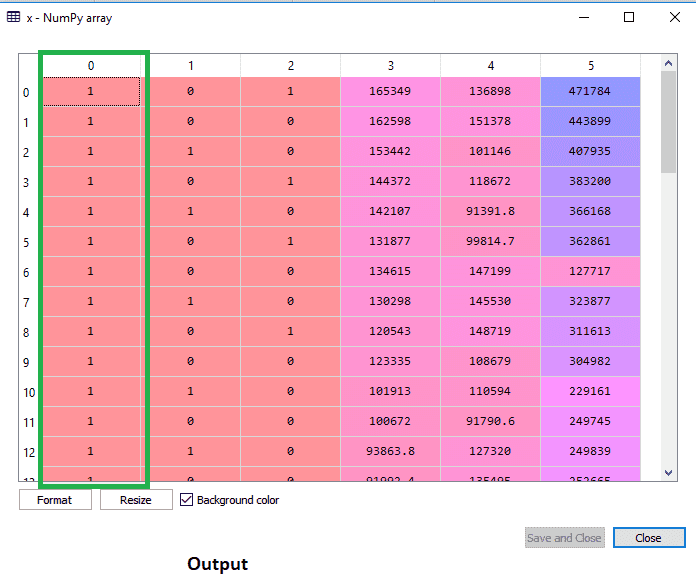The first column, which corresponds to the constant term of the MLR equation, is successfully added, as shown in the above output image.

## Step: 2:

• We are now going to use a backward elimination procedure. To begin, we'll establish a new feature vector called x_opt that only contains a collection of independent characteristics that have a significant impact on the dependent variable.
• Following that, we must set a significant level (0.5) and fit the model with all feasible predictors using the Backward Elimination method. So, to fit the model, we'll make a regressor_OLS object using the statsmodels library's new class OLS. Then we'll use the fit() technique to make it fit.
• Next, we'll need to compare the p-value to the SL value, therefore we'll utilize the summary() method to acquire a summary table of all the data. The code for it is as follows:

``````
x_opt = x [:, [0,1,2,3,4,5]]
regressor_OLS = sm.OLS(endog = y, exog = x_opt).fit()
regressor_OLS.summary()
```
```

Output: We will get a summary table by executing the above lines of code. Consider the below image: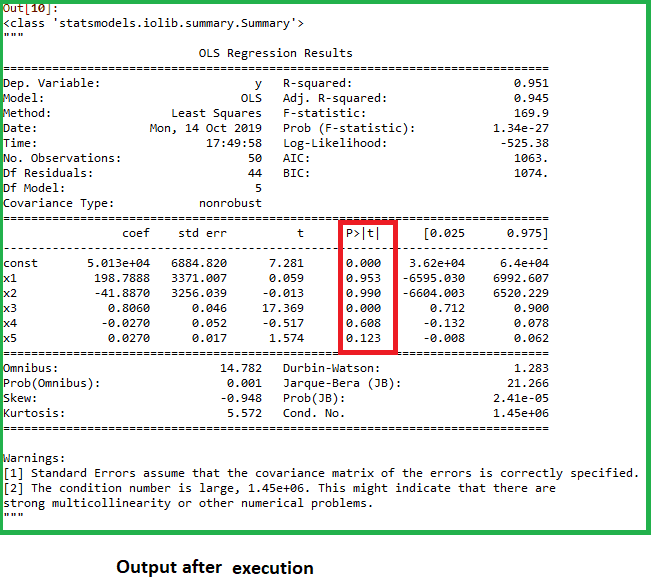The p-values of all the variables are plainly visible in the above graphic. Here, x1 and x2 are dummy variables, R&D cost is x3, Administration is x4, and Marketing is x5.
We'll pick the highest p-value from the table, which is for x1=0.953. We will delete the x1 variable (dummy variable) from the table and retrain the model now that we have the highest p-value that is bigger than the SL value. The code for it is as follows:

``````
x_opt = x[:, [0,2,3,4,5]]
regressor_OLS = sm.OLS(endog = y, exog = x_opt).fit()
regressor_OLS.summary()
```
```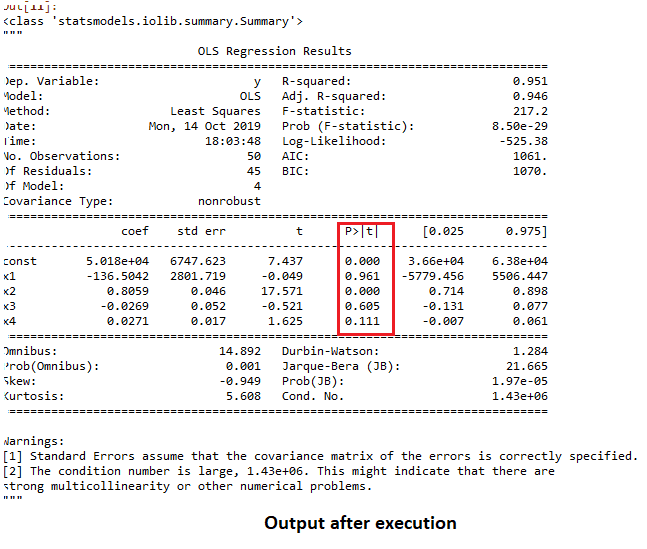Five variables remain, as shown in the output image. The greatest p-value in these variables is 0.961. As a result, we'll get rid of it in the next iteration.

• The greatest value now is 0.961 for the x1 variable, which is a dummy variable. As a result, we'll take it out and reassemble the model. The code for it is as follows:

``````
x_opt = x[:, [0,3,4,5]]
regressor_OLS = sm.OLS(endog = y, exog = x_opt).fit()
regressor_OLS.summary()
```
```

Output: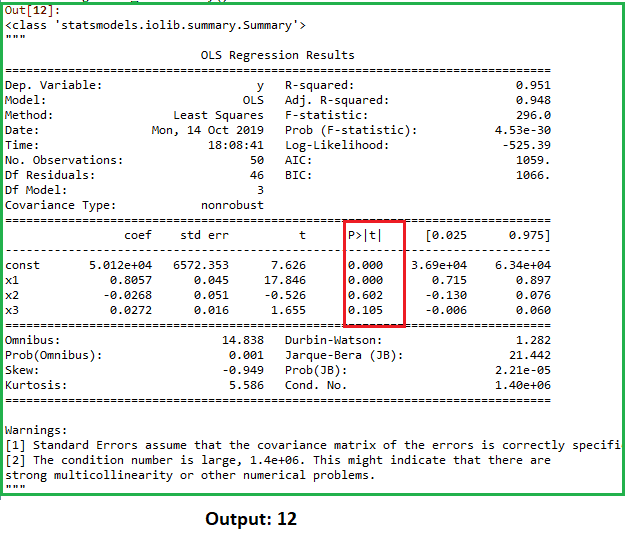The dummy variable(x2) has been deleted from the above output image. And the next highest value is.602, which is still higher than.5, thus it must be eliminated.

• Now we'll get rid of the Admin spend that's been accumulating. Refit the model using a 602 p-value.
``````
x_opt = x[:, [0,3,4,5]]
regressor_OLS = sm.OLS(endog = y, exog = x_opt).fit()
regressor_OLS.summary()
```
```

Output: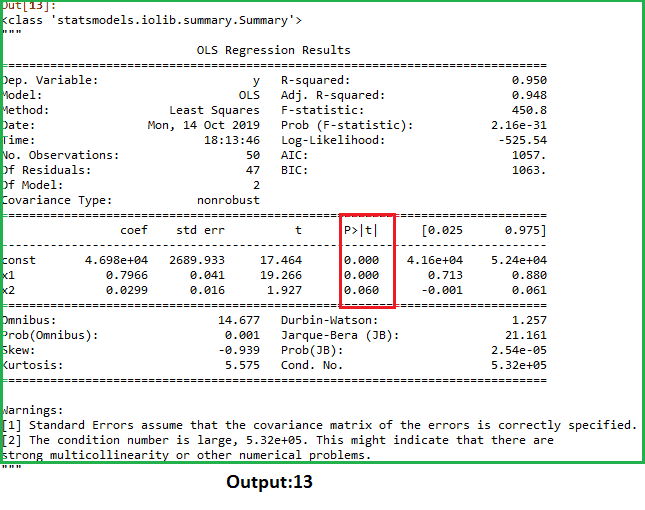The dummy variable(x2) has been eliminated as seen in the above output graphic. The next highest value is.602, which is still more than.5, thus it must be eliminated.

• Now we'll get rid of the Admin expense. Refit the model using the 602 p-value

``````
x_opt = x[:, [0,3,5]]
regressor_OLS=sm.OLS(endog = y, exog = x_opt).fit()
regressor_OLS.summary()
```
```

Output: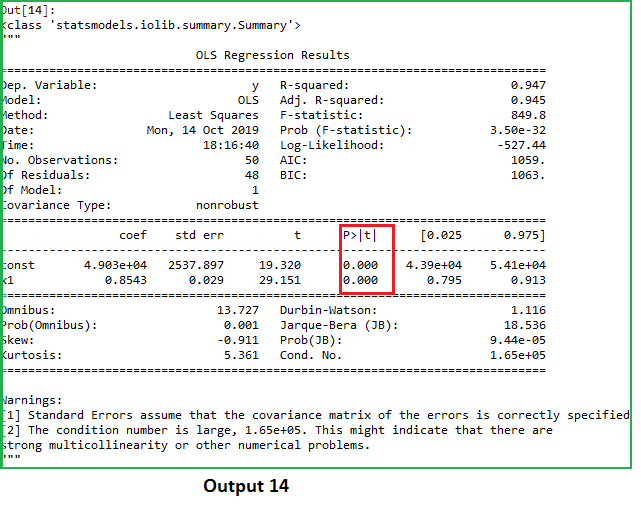Only two variables remain, as shown in the result image above. As a result, only the R&D independent variable is a predictive predictor. As a result, we can now accurately predict using this variable.

## Estimating the performance:

When we applied all of the features variables in the previous topic, we calculated the train and test score of the model. We'll now examine the score using only one feature variable (R&D spend). Now, here's what our data looks like: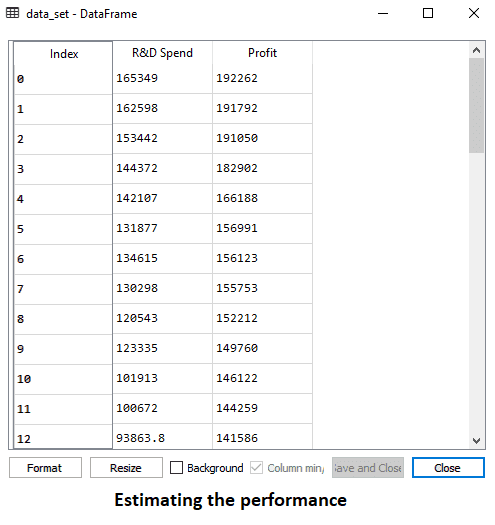Below is the code for Building Multiple Linear Regression model by only using R&D spend:

``````
#importing libraries
import numpy as nm
import matplotlib.pyplot as mtp
import pandas as pd

#importing datasets

#Extracting Independent and dependent Variable
x_BE = data_set.iloc[:, :-1].values
y_BE = data_set.iloc[:, 1].values

#Splitting the dataset into training and test set.
from sklearn.model_selection import train_test_split
x_BE_train, x_BE_test, y_BE_train, y_BE_test = train_test_split(x_BE, y_BE, test_size= 0.2, random_state=0)

#Fitting the MLR model to the training set:
from sklearn.linear_model import LinearRegression
regressor= LinearRegression()
regressor.fit(nm.array(x_BE_train).reshape(-1,1), y_BE_train)

#Predicting the Test set result;
y_pred = regressor.predict(x_BE_test)

#Cheking the score
print('Train Score: ', regressor.score(x_BE_train, y_BE_train))
print('Test Score: ', regressor.score(x_BE_test, y_BE_test))
```
```

Output:

After executing the above code, we will get the Training and test scores as:

``````
Train Score: 0.9449589778363044
Test Score: 0.9464587607787219
```
```

The training score is 94% accurate, and the test score is equally 94% accurate, as can be shown. There is a.00149 difference between the two scores. This number is quite similar to the previous value, 0.0154, where all variables were included.

Instead of using four variables, we just used one independent variable (R&D spend). As a result, our model is now both simple and accurate.